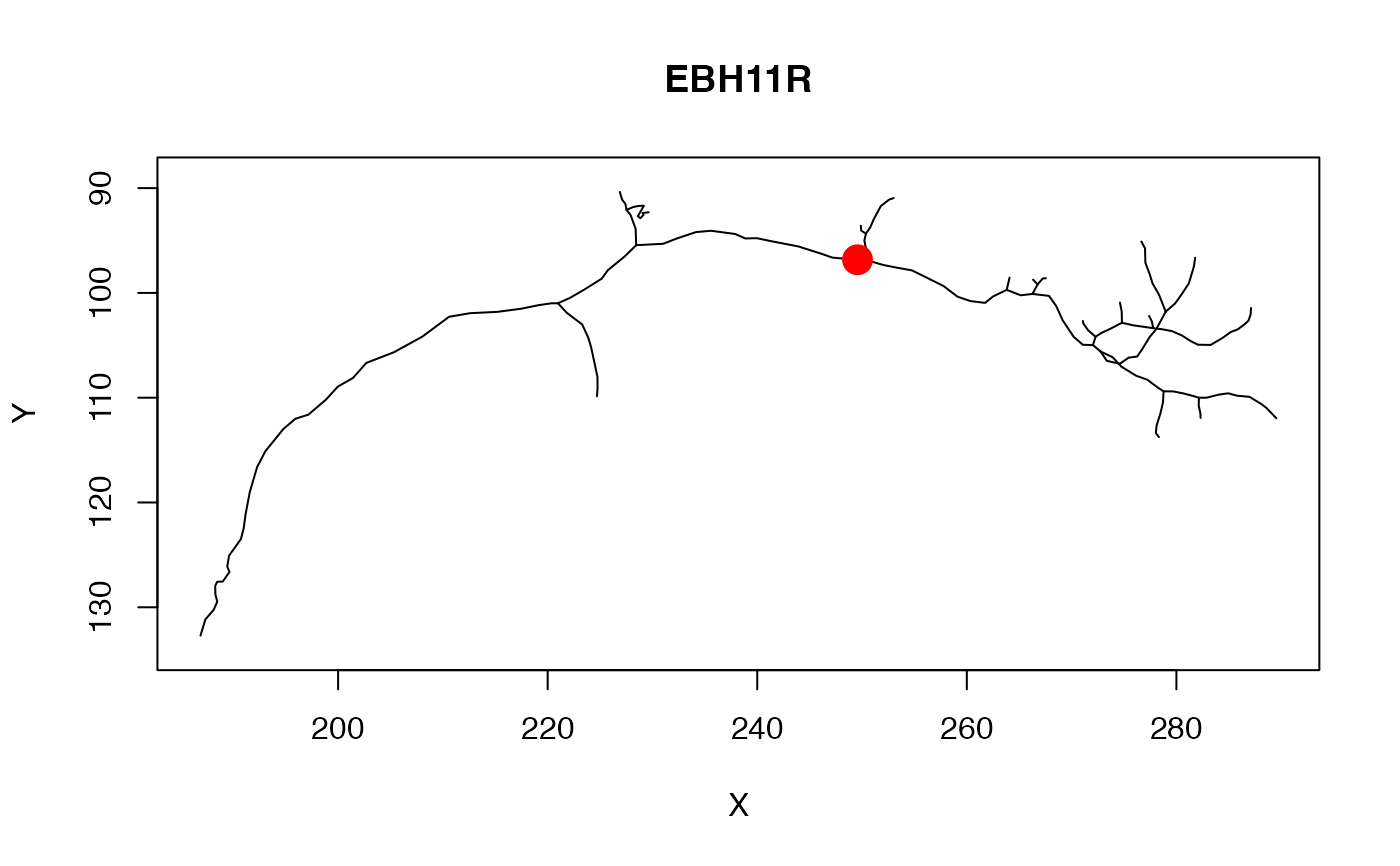intersect_plane.neuron finds the place where a neuron intersection

intersect_plane(x, plane, ...)

# S3 method for default
intersect_plane(x, plane, ...)

# S3 method for neuron
intersect_plane(x, plane, closestpoint = NULL, ...)

## Arguments

x

A neuron, set of line segments or other data - see details.

plane

A plane, specified by the 4 coefficients of the plane equation (see plane_coefficients)

...

closestpoint

Used to define the closest hit when there are multiple

## Value

A Nx3 matrix of the X,Y,Z positions of the intersections (NA when there is no intersection)

Other geometry: plane_coefficients()

## Examples

## Find plane coefficients
# point on plane
cent=c(250.4987, 95.73561, 140.2052)
# vector normal to plane
vec=c(0.7709581, 0.03417276, -0.411977)
plc=plane_coefficients(cent, vec)

## intersect with plane
ip=intersect_plane(Cell07PNs[], plc)
plot(Cell07PNs[], WithNodes=FALSE)
points(ip, ip, pch=19, cex=2, col='red')if (FALSE) {
plot3d(Cell07PNs[], col='grey', WithNodes=FALSE)